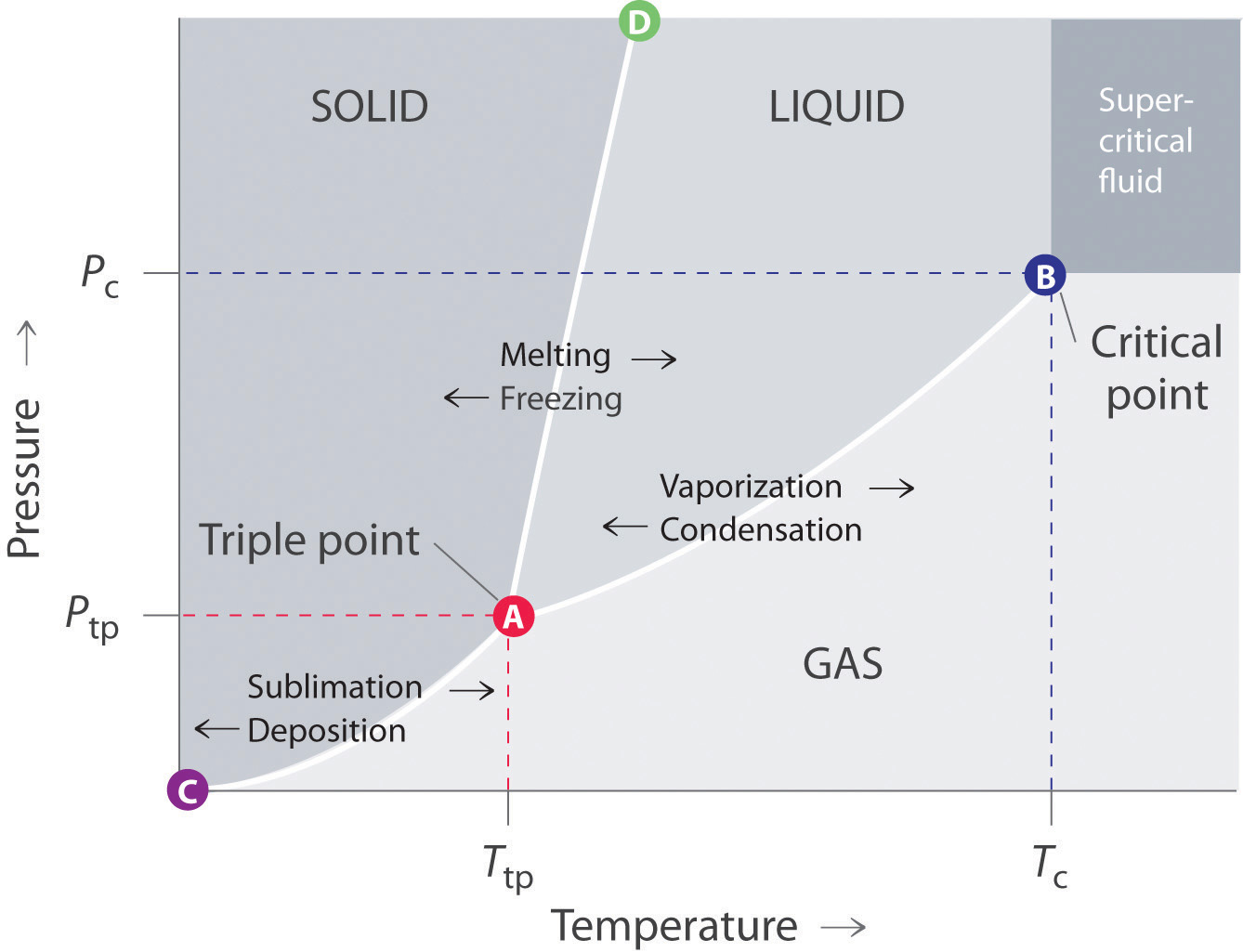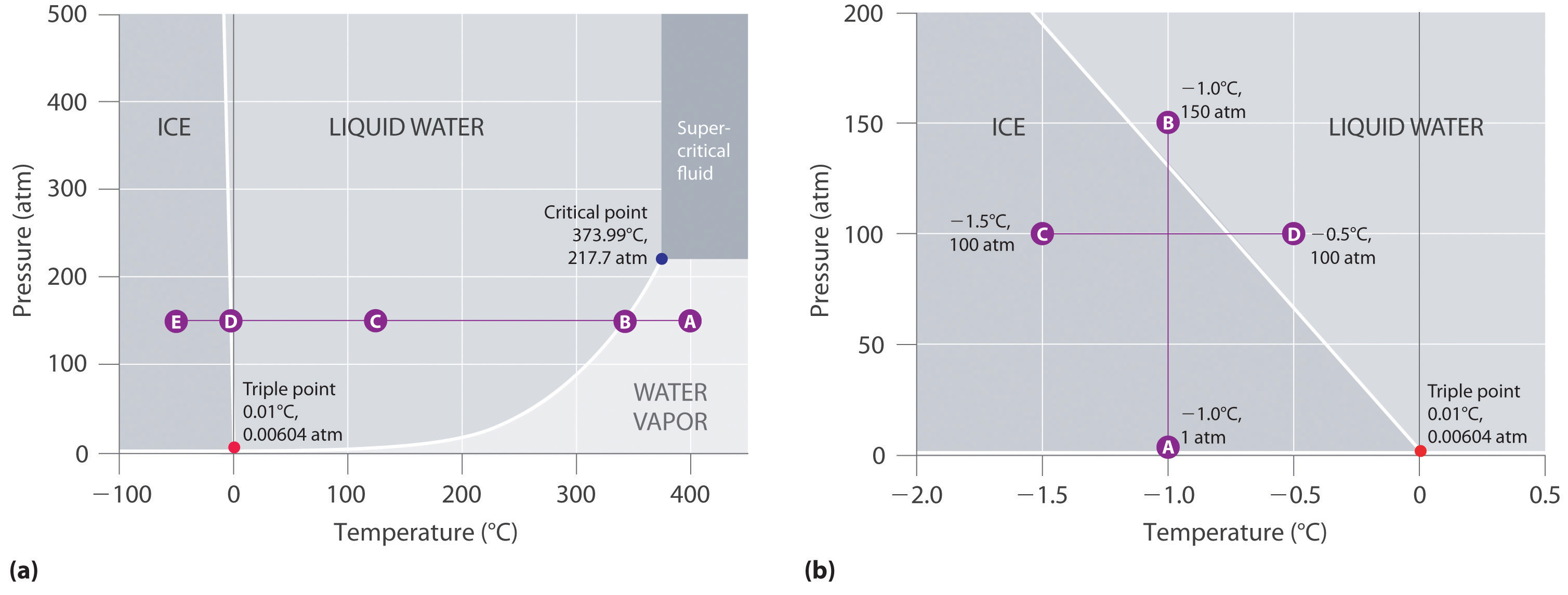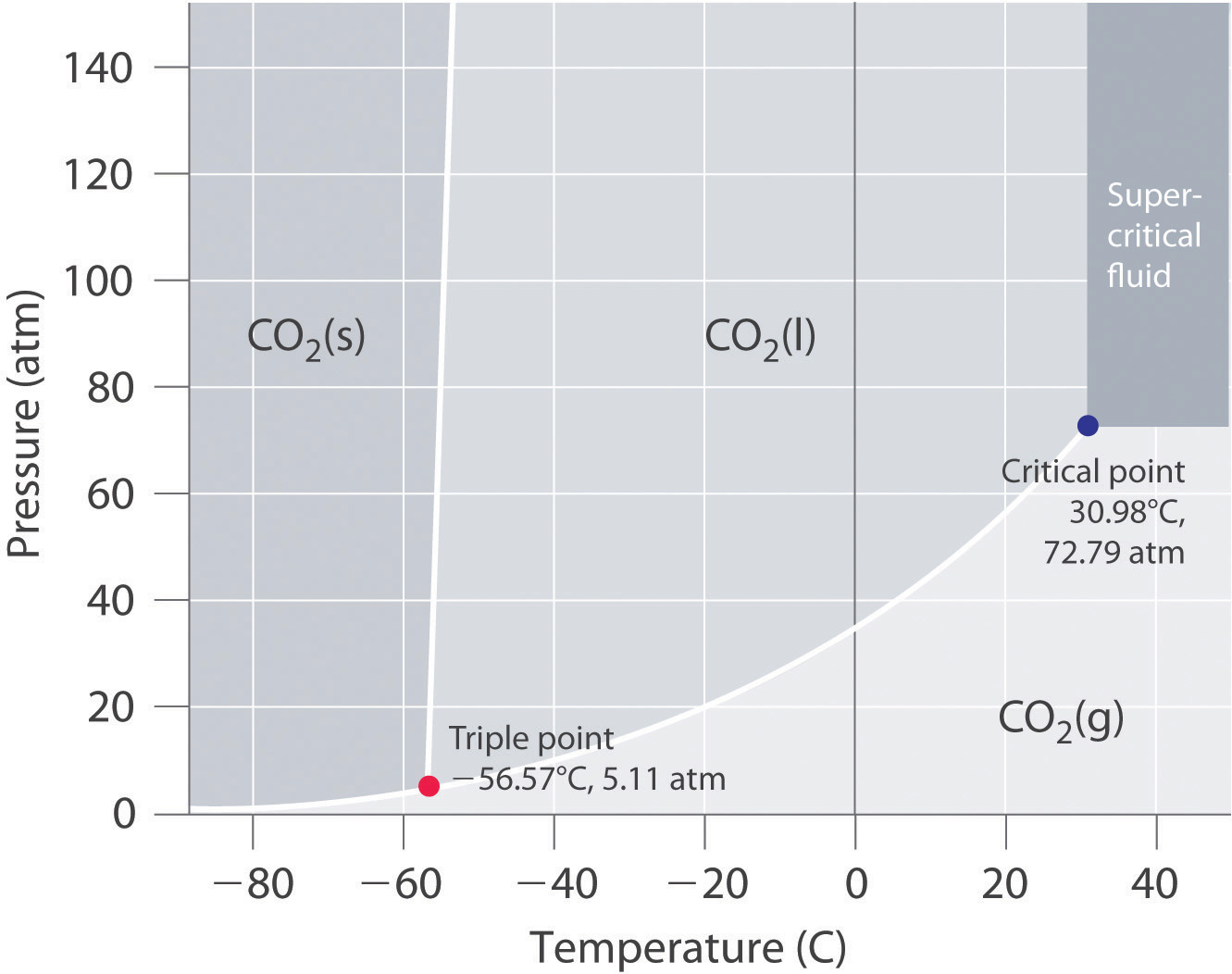This is “Phase Diagrams”, section 11.7 from the book Principles of General Chemistry (v. 1.0M). For details on it (including licensing), click here.

For more information on the source of this book, or why it is available for free, please see the project's home page. You can browse or download additional books there. To download a .zip file containing this book to use offline, simply click here.

Has this book helped you? Consider passing it on:
Creative Commons supports free culture from music to education. Their licenses helped make this book available to you.
DonorsChoose.org helps people like you help teachers fund their classroom projects, from art supplies to books to calculators.

## 11.7 Phase Diagrams

### Learning Objective

1. To understand the general features of a phase diagram.

The state exhibited by a given sample of matter depends on the identity, temperature, and pressure of the sample. A phase diagramA graphic summary of the physical state of a substance as a function of temperature and pressure in a closed system. is a graphic summary of the physical state of a substance as a function of temperature and pressure in a closed system.

A typical phase diagram consists of discrete regions that represent the different phases exhibited by a substance (Figure 11.22 "A Typical Phase Diagram for a Substance That Exhibits Three Phases—Solid, Liquid, and Gas—and a Supercritical Region"). Each region corresponds to the range of combinations of temperature and pressure over which that phase is stable. The combination of high pressure and low temperature (upper left of Figure 11.22 "A Typical Phase Diagram for a Substance That Exhibits Three Phases—Solid, Liquid, and Gas—and a Supercritical Region") corresponds to the solid phase, whereas the gas phase is favored at high temperature and low pressure (lower right). The combination of high temperature and high pressure (upper right) corresponds to a supercritical fluid.

Figure 11.22 A Typical Phase Diagram for a Substance That Exhibits Three Phases—Solid, Liquid, and Gas—and a Supercritical Region### Note the Pattern

The solid phase is favored at low temperature and high pressure; the gas phase is favored at high temperature and low pressure.

## General Features of a Phase Diagram

The lines in a phase diagram correspond to the combinations of temperature and pressure at which two phases can coexist in equilibrium. In Figure 11.22 "A Typical Phase Diagram for a Substance That Exhibits Three Phases—Solid, Liquid, and Gas—and a Supercritical Region", the line that connects points A and D separates the solid and liquid phases and shows how the melting point of a solid varies with pressure. The solid and liquid phases are in equilibrium all along this line; crossing the line horizontally corresponds to melting or freezing. The line that connects points A and B is the vapor pressure curve of the liquid, which we discussed in Section 11.4 "Vapor Pressure". It ends at the critical point, beyond which the substance exists as a supercritical fluid. The line that connects points A and C is the vapor pressure curve of the solid phase. Along this line, the solid is in equilibrium with the vapor phase through sublimation and deposition. Finally, point A, where the solid/liquid, liquid/gas, and solid/gas lines intersect, is the triple pointThe point in a phase diagram where the solid/liquid, liquid/gas, and solid/gas lines intersect; it represents the only combination of temperature and pressure at which all three phases are in equilibrium and can therefore exist simultaneously.; it is the only combination of temperature and pressure at which all three phases (solid, liquid, and gas) are in equilibrium and can therefore exist simultaneously. Because no more than three phases can ever coexist, a phase diagram can never have more than three lines intersecting at a single point.

Remember that a phase diagram, such as the one in Figure 11.22 "A Typical Phase Diagram for a Substance That Exhibits Three Phases—Solid, Liquid, and Gas—and a Supercritical Region", is for a single pure substance in a closed system, not for a liquid in an open beaker in contact with air at 1 atm pressure. In practice, however, the conclusions reached about the behavior of a substance in a closed system can usually be extrapolated to an open system without a great deal of error.

## The Phase Diagram of Water

Figure 11.23 "Two Versions of the Phase Diagram of Water" shows the phase diagram of water and illustrates that the triple point of water occurs at 0.01°C and 0.00604 atm (4.59 mmHg). Far more reproducible than the melting point of ice, which depends on the amount of dissolved air and the atmospheric pressure, the triple point (273.16 K) is used to define the absolute (Kelvin) temperature scale. The triple point also represents the lowest pressure at which a liquid phase can exist in equilibrium with the solid or vapor. At pressures less than 0.00604 atm, therefore, ice does not melt to a liquid as the temperature increases; the solid sublimes directly to water vapor. Sublimation of water at low temperature and pressure can be used to “freeze-dry” foods and beverages. The food or beverage is first cooled to subzero temperatures and placed in a container in which the pressure is maintained below 0.00604 atm. Then, as the temperature is increased, the water sublimes, leaving the dehydrated food (such as that used by backpackers or astronauts) or the powdered beverage (as with freeze-dried coffee).

The phase diagram for water illustrated in part (b) in Figure 11.23 "Two Versions of the Phase Diagram of Water" shows the boundary between ice and water on an expanded scale. The melting curve of ice slopes up and slightly to the left rather than up and to the right as in Figure 11.22 "A Typical Phase Diagram for a Substance That Exhibits Three Phases—Solid, Liquid, and Gas—and a Supercritical Region"; that is, the melting point of ice decreases with increasing pressure; at 100 MPa (987 atm), ice melts at −9°C. Water behaves this way because it is one of the few known substances for which the crystalline solid is less dense than the liquid (others include antimony and bismuth). Increasing the pressure of ice that is in equilibrium with water at 0°C and 1 atm tends to push some of the molecules closer together, thus decreasing the volume of the sample. The decrease in volume (and corresponding increase in density) is smaller for a solid or a liquid than for a gas, but it is sufficient to melt some of the ice.

Figure 11.23 Two Versions of the Phase Diagram of Water(a) In this graph with linear temperature and pressure axes, the boundary between ice and liquid water is almost vertical. (b) This graph with an expanded scale illustrates the decrease in melting point with increasing pressure. (The letters refer to points discussed in Example 10.)

In part (b) in Figure 11.23 "Two Versions of the Phase Diagram of Water", point A is located at P = 1 atm and T = −1.0°C, within the solid (ice) region of the phase diagram. As the pressure increases to 150 atm while the temperature remains the same, the line from point A crosses the ice/water boundary to point B, which lies in the liquid water region. Consequently, applying a pressure of 150 atm will melt ice at −1.0°C. We have already indicated that the pressure dependence of the melting point of water is of vital importance. If the solid/liquid boundary in the phase diagram of water were to slant up and to the right rather than to the left, ice would be denser than water, ice cubes would sink, water pipes would not burst when they freeze, and antifreeze would be unnecessary in automobile engines.

Until recently, many textbooks described ice skating as being possible because the pressure generated by the skater’s blade is high enough to melt the ice under the blade, thereby creating a lubricating layer of liquid water that enables the blade to slide across the ice. Although this explanation is intuitively satisfying, it is incorrect, as we can show by a simple calculation. Recall from Chapter 10 "Gases" that pressure (P) is the force (F) applied per unit area (A):

Equation 11.4

$P = F A$

To calculate the pressure an ice skater exerts on the ice, we need to calculate only the force exerted and the area of the skate blade. If we assume a 75.0 kg (165 lb) skater, then the force exerted by the skater on the ice due to gravity is

Equation 11.5

F = mg

where m is the mass and g is the acceleration due to Earth’s gravity (9.81 m/s2). Thus the force is

Equation 11.6

F = (75.0 kg)(9.81 m/s2) = 736 (kg·m)/s2 = 736 N

If we assume that the skate blades are 2.0 mm wide and 25 cm long, then the area of the bottom of each blade is

Equation 11.7

A = (2.0 × 10−3 m)(25 × 10−2 m) = 5.0 × 10−4 m2

If the skater is gliding on one foot, the pressure exerted on the ice is

Equation 11.8

The pressure is much lower than the pressure needed to decrease the melting point of ice by even 1°C, and experience indicates that it is possible to skate even when the temperature is well below freezing. Thus pressure-induced melting of the ice cannot explain the low friction that enables skaters (and hockey pucks) to glide. Recent research indicates that the surface of ice, where the ordered array of water molecules meets the air, consists of one or more layers of almost liquid water. These layers, together with melting induced by friction as a skater pushes forward, appear to account for both the ease with which a skater glides and the fact that skating becomes more difficult below about −7°C, when the number of lubricating surface water layers decreases.

## The Phase Diagram of Carbon Dioxide

In contrast to the phase diagram of water, the phase diagram of CO2 (Figure 11.24 "The Phase Diagram of Carbon Dioxide") has a more typical melting curve, sloping up and to the right. The triple point is −56.6°C and 5.11 atm, which means that liquid CO2 cannot exist at pressures lower than 5.11 atm. At 1 atm, therefore, solid CO2 sublimes directly to the vapor while maintaining a temperature of −78.5°C, the normal sublimation temperature. Solid CO2 is generally known as dry ice because it is a cold solid with no liquid phase observed when it is warmed. Also notice the critical point at 30.98°C and 72.79 atm. In addition to the uses discussed in Section 11.6 "Critical Temperature and Pressure", supercritical carbon dioxide is emerging as a natural refrigerant, making it a low carbon (and thus a more environmentally friendly) solution for domestic heat pumps.

Figure 11.24 The Phase Diagram of Carbon DioxideNote the critical point, the triple point, and the normal sublimation temperature in this diagram.

### Example 10

Referring to the phase diagram of water in Figure 11.23 "Two Versions of the Phase Diagram of Water",

1. predict the physical form of a sample of water at 400°C and 150 atm.
2. describe the changes that occur as the sample in part (a) is slowly allowed to cool to −50°C at a constant pressure of 150 atm.

Given: phase diagram, temperature, and pressure

Asked for: physical form and physical changes

Strategy:

A Identify the region of the phase diagram corresponding to the initial conditions and identify the phase that exists in this region.

B Draw a line corresponding to the given pressure. Move along that line in the appropriate direction (in this case cooling) and describe the phase changes.

Solution:

1. A Locate the starting point on the phase diagram in part (a) in Figure 11.23 "Two Versions of the Phase Diagram of Water". The initial conditions correspond to point A, which lies in the region of the phase diagram representing water vapor. Thus water at T = 400°C and P = 150 atm is a gas.
2. B Cooling the sample at constant pressure corresponds to moving left along the horizontal line in part (a) in Figure 11.23 "Two Versions of the Phase Diagram of Water". At about 340°C (point B), we cross the vapor pressure curve, at which point water vapor will begin to condense and the sample will consist of a mixture of vapor and liquid. When all of the vapor has condensed, the temperature drops further, and we enter the region corresponding to liquid water (indicated by point C). Further cooling brings us to the melting curve, the line that separates the liquid and solid phases at a little below 0°C (point D), at which point the sample will consist of a mixture of liquid and solid water (ice). When all of the water has frozen, cooling the sample to −50°C takes us along the horizontal line to point E, which lies within the region corresponding to solid water. At P = 150 atm and T = −50°C, therefore, the sample is solid ice.

Exercise

Referring to the phase diagram of water in Figure 11.23 "Two Versions of the Phase Diagram of Water", predict the physical form of a sample of water at −0.0050°C as the pressure is gradually increased from 1.0 mmHg to 218 atm.

Answer: The sample is initially a gas, condenses to a solid as the pressure increases, and then melts when the pressure is increased further to give a liquid.

### Summary

The states of matter exhibited by a substance under different temperatures and pressures can be summarized graphically in a phase diagram, which is a plot of pressure versus temperature. Phase diagrams contain discrete regions corresponding to the solid, liquid, and gas phases. The solid and liquid regions are separated by the melting curve of the substance, and the liquid and gas regions are separated by its vapor pressure curve, which ends at the critical point. Within a given region, only a single phase is stable, but along the lines that separate the regions, two phases are in equilibrium at a given temperature and pressure. The lines separating the three phases intersect at a single point, the triple point, which is the only combination of temperature and pressure at which all three phases can coexist in equilibrium. Water has an unusual phase diagram: its melting point decreases with increasing pressure because ice is less dense than liquid water. The phase diagram of carbon dioxide shows that liquid carbon dioxide cannot exist at atmospheric pressure. Consequently, solid carbon dioxide sublimes directly to a gas.

### Key Takeaway

• A phase diagram is a graphic summary of the physical state of a substance as a function of temperature and pressure in a closed system. It shows the triple point, the critical point, and four regions: solid, liquid, gas, and a supercritical region.

### Conceptual Problems

1. A phase diagram is a graphic representation of the stable phase of a substance at any combination of temperature and pressure. What do the lines separating different regions in a phase diagram indicate? What information does the slope of a line in a phase diagram convey about the physical properties of the phases it separates? Can a phase diagram have more than one point where three lines intersect?

2. If the slope of the line corresponding to the solid/liquid boundary in the phase diagram of water were positive rather than negative, what would be the effect on aquatic life during periods of subzero temperatures? Explain your answer.

### Answer

1. The lines in a phase diagram represent boundaries between different phases; at any combination of temperature and pressure that lies on a line, two phases are in equilibrium. It is physically impossible for more than three phases to coexist at any combination of temperature and pressure, but in principle there can be more than one triple point in a phase diagram. The slope of the line separating two phases depends upon their relative densities. For example, if the solid–liquid line slopes up and to the right, the liquid is less dense than the solid, while if it slopes up and to the left, the liquid is denser than the solid.

### Numerical Problems

1. Naphthalene (C10H8) is the key ingredient in mothballs. It has normal melting and boiling points of 81°C and 218°C, respectively. The triple point of naphthalene is 80°C at 1000 Pa. Use these data to construct a phase diagram for naphthalene and label all the regions of your diagram.

2. Argon is an inert gas used in welding. It has normal boiling and freezing points of 87.3 K and 83.8 K, respectively. The triple point of argon is 83.8 K at 0.68 atm. Use these data to construct a phase diagram for argon and label all the regions of your diagram.